Plots and Results, Lepton-Photon 2003

Standard input constraints:

 Inputs to the standard global fit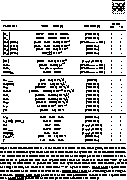ps Numerical results the standard global fit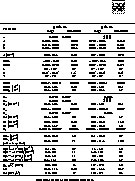ps Confidence levels in the large (rhobar,etabar) plane obtained from the global fit. The constraint from the WA sin2beta/phi1 (from charmoniom modes - see average from HFAG) is overlaid.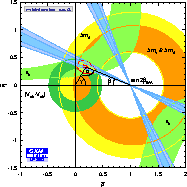eps Same plot as above, without the constraint of sin2beta overlaid.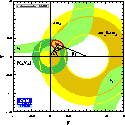eps Confidence levels in the large (rhobar,etabar) plane obtained from the global fit. The constraint from the WA sin2beta/phi1 (from charmoniom modes - see average from HFAG) is included in the fit.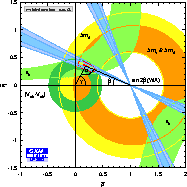eps Same plot as above, without the constraint of sin2beta overlaid.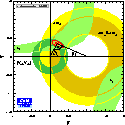eps Confidence levels in the large (rhobar,etabar) plane obtained from the global fit. The constraint from the WA sin2beta/phi1 (from all modes - see average from HFAG) is overlaid.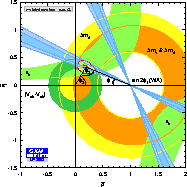eps Confidence levels in the large (rhobar,etabar) plane obtained from the global fit. The constraint from the WA sin2beta/phi1 (from all modes - see average from HFAG) is included in the fit.eps Confidence levels in the large (rhobar,etabar) plane obtained from the global fit. The constraints from sin2beta/phi1 from charmonium modes and from b->s penguin modes are overlaid - see HFAG.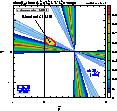eps Confidence levels in the large (rhobar,etabar) plane obtained from the global fit. The constraints from sin2beta/phi1 from charmonium modes and from B->phiKs are overlaid - see HFAG.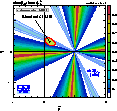eps Constraint in the rho-eta plane obtained from B->D*pi (measuring sin(2beta+gamma) [sin(2phi1+phi3)]), see HFAG.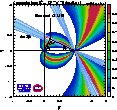eps Constraint in the rho-eta plane obtained from B->D0 K (measuring gamma [phi3]), see HFAG.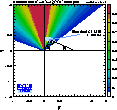eps

Charmless decays constraints:

 B->pi+pi- penguin to tree ratio: using as input the measured Cpp and Spp coefficients (see HFAG) together with the Wolfenstein parameters rhobar and etabar from the Global CKM fit using standard constraints, one can infer module and phase of the complex penguin to tree (P/T) ratio in B-> pi+pi- decays within the Standard Model. This plot shows the confidence level representation of the P/T phase versus its module.eps Constraining alpha/phi2: using as input the measured Cpp and Spp coefficients together with the present pipi branching fractions of all charges and B flavors including the newly discovered pi0pi0 (see HFAG), one can perform various numerical analyses aiming at a constraint on alpha/phi2. The Gronau-London SU(2) analysis (electroweak penguins and other SU(2)-breaking sources are neglected here). Assuming SU(3) symmetry, one can identify the module of the penguin occurring in the pi+pi- amplitude with the Cabibbo-enhanced penguin in Bd decays to K+pi- No SU(3)-breaking corrections are applied here. Assuming SU(3) symmetry, one can identify the module of the penguin occurring in the pi+pi- amplitude with the penguin decay of a B+ to K0pi+. First order SU(3)-breaking corrections have been applied here. In contrast to the phenomenological methods above, once can apply QCD Factorization (BBNS) predicting the complex penguin-to-tree ratio, to constrain a from the time-dependent CP measurement. The confidence levels obtained with these methods are shown in the right hand figure. It is compared to the constraint obtained from the global CKM fit using the standard constraints (including the HFAG average of sin(2beta).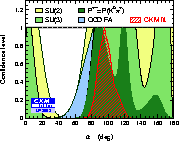eps Constraints in the Spipi-Cpipi plane within the frameworks of SU(2), SU(3), QCD Factorization (see HFAG).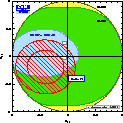eps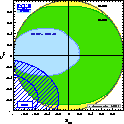eps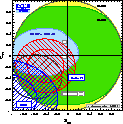eps Constraint in the rho-eta plane from B0->pi+pi- within the QCD factorization framework. The average BABAR and Belle results (seeHFAG) are used.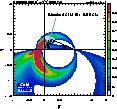eps